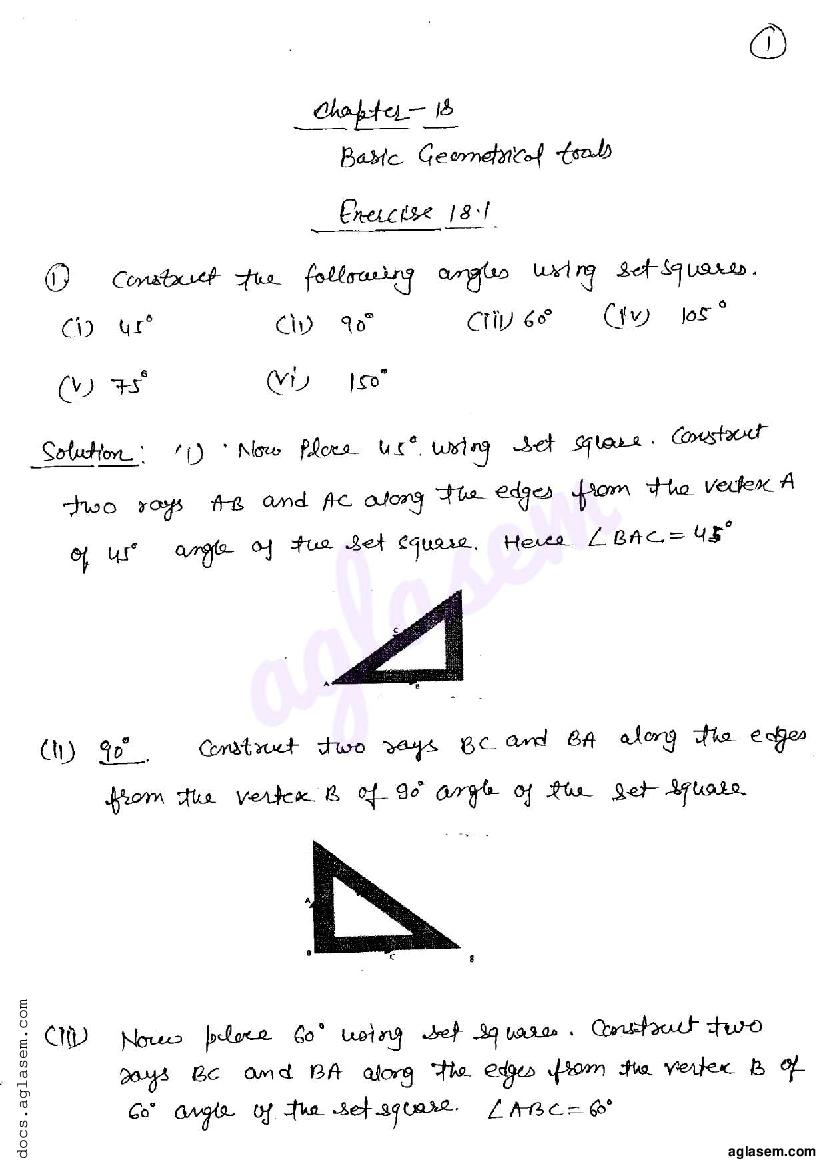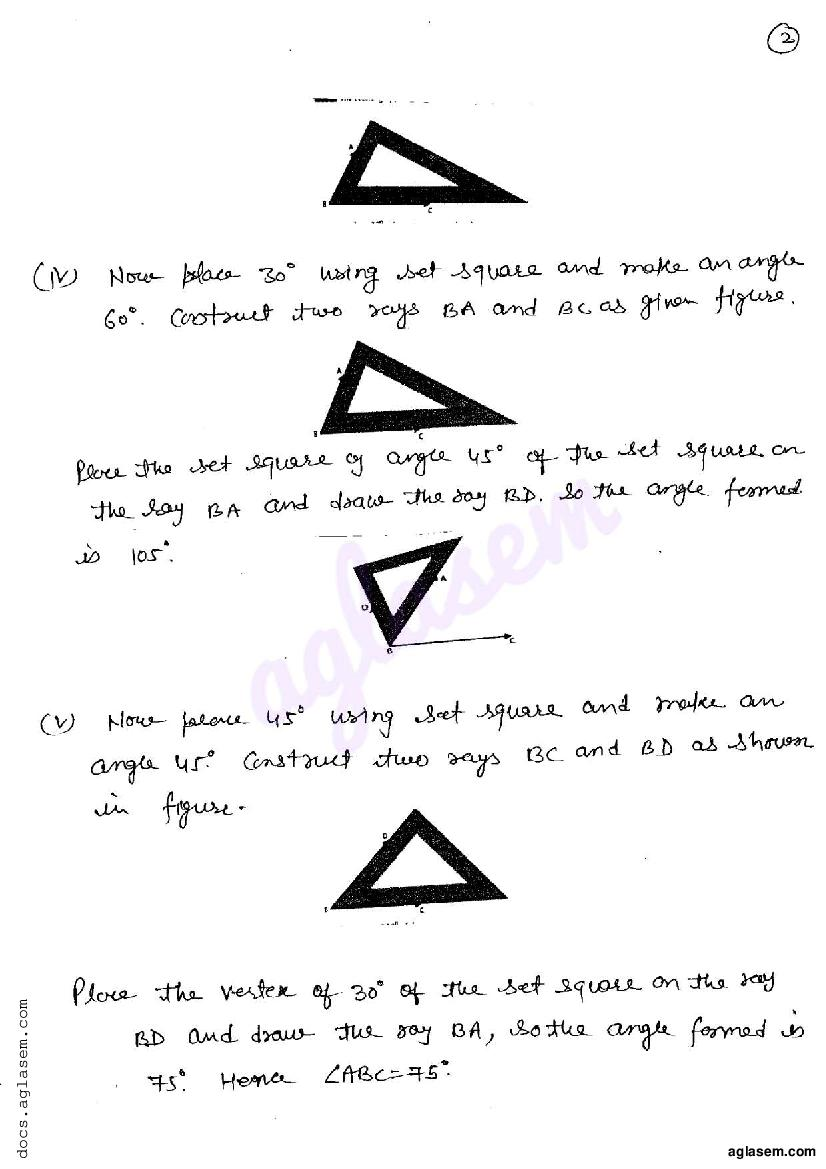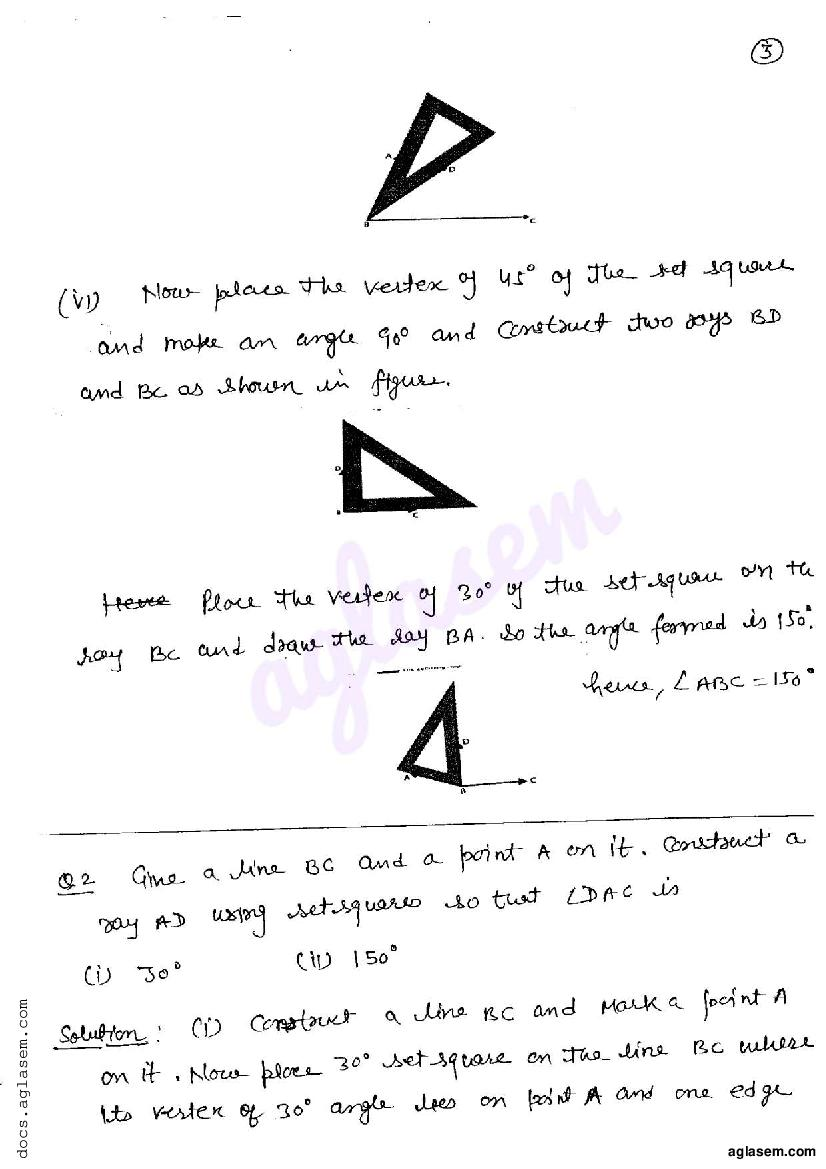# RD Sharma Solutions Class 6 Chapter 18 Basic Geometrical Tools Exercise 18.1

Here you can get free RD Sharma Solutions for Class 6 Maths Chapter 18 Basic Geometrical Tools Exercise 18.1. All RD Sharma Book Solutions are given here exercise wise for the chapter Basic Geometrical Tools. RD Sharma Solutions are helpful in the preparation of several school level, graduate and undergraduate level competitive exams. Practicing questions from RD Sharma Mathematics Solutions for Class 6 Chapter 18 Basic Geometrical Tools is proven to enhance your math skills.

 Class: Class 6th Chapter: Chapter 18 Exercise: Exercise 18.1 Name: Basic Geometrical Tools

## RD Sharma Solutions Class 6 Chapter 18 Basic Geometrical Tools Exercise 18.1

RD Sharma Class 6 Solutions Chapter 18 for Basic Geometrical Tools Exercise 18.1 are given below.

RD Sharma Solutions Class 6 Maths Chapter 18 Basic Geometrical Tools Exercise 18.1